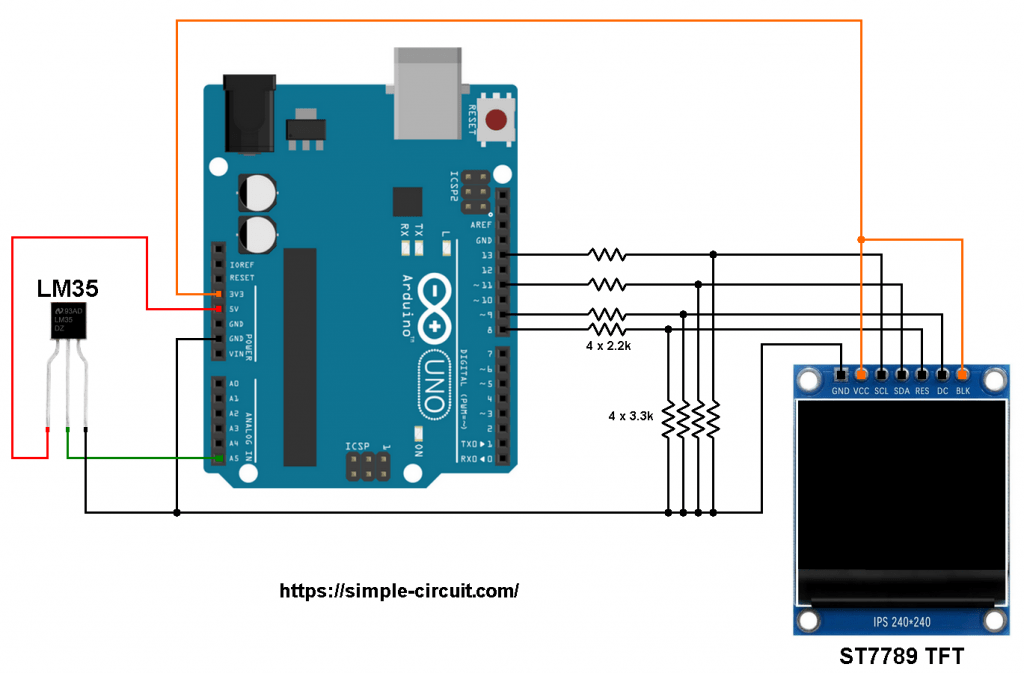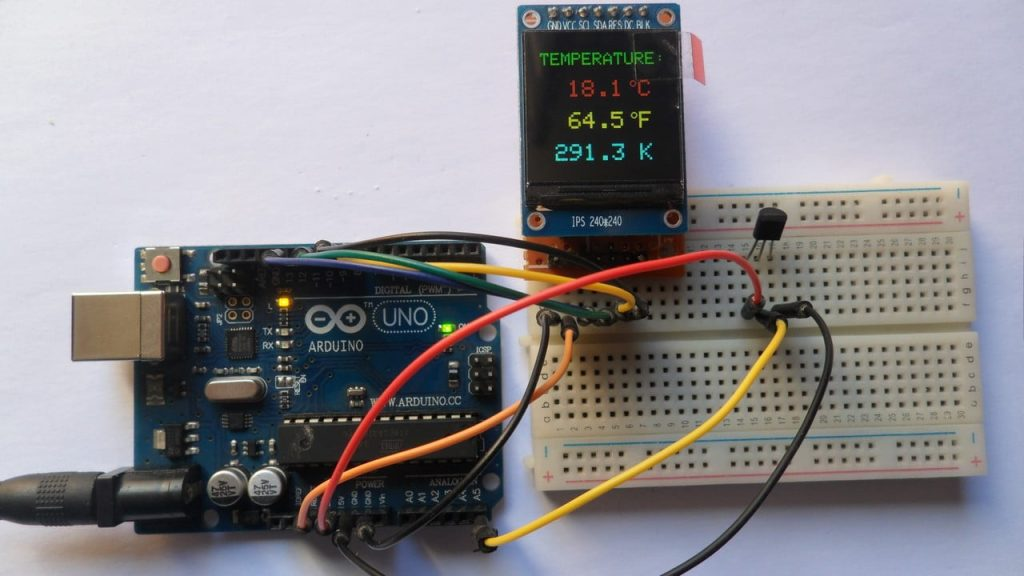# Arduino Digital Thermometer with ST7789 TFT and LM35 Sensor

This Arduino project shows how to build a digital thermometer using LM35 analog temperature sensor where temperature value is printed on ST7789 TFT (in degrees Celsius, Kelvin and degrees Fahrenheit).
The ST7789 TFT module contains a display controller with the same name: ST7789. It’s a color display that uses SPI interface protocol and requires 3, 4 or 5 control pins, it’s low cost and easy to use. This display is an IPS display, it comes in different sizes (1.3″, 1.54″ …) but all of them should have the same resolution of 240×240 pixel, this means it has 57600 pixels. This module works with 3.3V only and it doesn’t support 5V (not 5V tolerant).

TFT: Thin-Film Transistor.
SPI: Serial Peripheral Interface.
IPS: In-Plane Switching.

To see how to interface Arduino with ST7789 TFT display, visit this post:
Interfacing Arduino with ST7789 TFT Display – Graphics Test Example

The LM35 temperature sensor is a three pin device (VCC, OUT and GND) with an output voltage linearly related to Centigrade temperature. Since the LM35 output varies with dependent to the temperature, we need an ADC (Analog-to-Digital Converter) module to measure this voltage. The NodeMCU microcontroller (ESP8266EX) has one ADC module with 10-bit resolution.

The LM35 output has linear +10mV/°C scale factor means the following:
If the output voltage =   10mV —> temperature =   1°C
If the output voltage = 100mV —> temperature = 10°C
If the output voltage = 200mV —> temperature = 20°C
If the output voltage = 370mV —> temperature = 37°C
and so on.

LM35 Futures (from datasheet):

• Calibrated Directly in ° Celsius (Centigrade)
• Linear + 10 mV/°C Scale Factor
• 0.5°C Ensured Accuracy (at +25°C)
• Rated for Full −55°C to +150°C Range
• Suitable for Remote Applications
• Low Cost Due to Wafer-Level Trimming
• Operates from 4 to 30 V
• Less than 60-μA Current Drain
• Low Self-Heating, 0.08°C in Still Air
• Nonlinearity Only ±¼°C Typical
• Low Impedance Output, 0.1 Ω for 1 mA Load

The ADC module converts analog data into digital data. The ATmega328P MCU has a 10-bit ADC module and a built-in reference voltage of 1.1V. Using the reference voltage should give better results.
Normally negative and positive references of the ADC module are VSS and VDD respectively, but we don’t know if VDD is exactly equal to 5.00V (at any time), hence we should use the reference voltage (1.1V) as a positive reference of the ADC module.

Hardware Required:

• Arduino board
• ST7789 TFT display module (1.3″, 1.54″ …)
• LM35 temperature sensor   —->   datasheet
• 4 x 3.3k ohm resistor (+1 if the display module has CS pin)
• 4 x 2.2k ohm resistor (+1 if the display module has CS pin)
• Jumper wires

Arduino with ST7789 TFT and LM35 sensor circuit:
The image below shows project circuit schematic diagram.

The LM35 sensor has 3 pins (from left to right):
Pin 1 is power supply pin, connected to Arduino 5V pin,
Pin 2: output pin, connected to Arduino analog pin 5 (A5),
Pin 3: GND (ground), connected to Arduino GND pin.The ST7789 display module shown in project circuit diagram has 7 pins: (from right to left): GND (ground), VCC, SCL (serial clock), SDA (serial data), RES (reset), DC (or D/C: data/command) and BLK (back light).
Connecting the BLK pin is optional. The back light turns off when the BLK pin connected to the ground (GND).

As mentioned above, the ST7789 TFT display controller works with 3.3V only (power supply and control lines). The display module is supplied with 3.3V (between VCC and GND) which comes from the Arduino board.

All Arduino UNO board output pins are 5V, connecting a 5V pin to the ST7789 TFT display may damage its controller.
To connect the Arduino to the display module, I used voltage divider for each line which means there are 4 voltage dividers. Each voltage divider consists of 2.2k and 3.3k resistors, this drops the 5V into 3V which is sufficient.

If the display module has a CS pin (Chip Select) then it should be connected to Arduino digital pin 10 through another voltage divider.

So, the ST7789 TFT display is connected to the Arduino board as follows (each one through voltage divider):
RST pin is connected to Arduino digital pin 8,
DC pin is connected to Arduino digital pin 9,
SDA pin is connected to Arduino digital pin 11,
SCL pin is connected to Arduino digital pin 13.

Other pins are connected as follows:
VCC pin is connected to Arduino 3V3 pin,
GND pin is connected to Arduino GND pin,
BL (LED) pin is connected to Arduino 3V3 pin (optional).

Arduino with ST7789 TFT and LM35 sensor code:
The following Arduino code requires 2 libraries from Adafruit Industries:
The first library is a driver for the ST7789 TFT display which can be installed from Arduino IDE library manager (Sketch —> Include Library —> Manage Libraries …, in the search box write “st7789” and install the one from Adafruit).

The second library is Adafruit graphics library which can be installed also from Arduino IDE library manager.

The same thing for other library file.

Hints:
The libraries are included in the main code as follows:

The ST7789 TFT module pins (CS, RST and DC) connections are defined as shown below (even the display module has no CS pin but its definition is required by the Adafruit ST7789 library):

The other display pins (SDA and SCL) are connected to Arduino hardware SPI module pins (digital pin 11 and digital pin 13 respectively for MOSI and SCLK).

The Adafruit ST7789 library is initialized with this line:

And the TFT display is initialized using the following command:

Reading voltage quantity using the ADC module gives a number between 0 and 1023 (10-bit resolution), 0V is represented by 0 and 1.1V is represented by 1023 (ADC positive reference is 1.1V) . Converting back the ADC digital value is easy, we can use the following equation:
Multiplying the previous result by 100 (LM35 scale factor is 10mV/°C = 0.01V/°C) gives the actual temperature:
where: 0.1075 = 100 * 1.1 / 1023  and  9.3 = 1 / 0.1075
To use the internal 1.1V reference I used the command: analogReference(INTERNAL);

In this example I used one number after the decimal point, multiplying the temperature by 10 gives the temperature in tenths degree Celsius (output value of “274” equals 27.4 °Celsius), so the final result is:

The temperature in tenths degree Fahrenheit =  (tenth °Celsius) x 9/5 +320 (because: °F = °Cx9/5 + 32) and the temperature in tenths Kelvin = (tenth °Celsius) + 2732 (because: K = °C + 273.16).

To get the actual value of each quantity we’ve to divide it by 10. The line below shows an example for temperature in Kelvin:

We get the first 3 digits by dividing the tenths value by 10, and the tenths number (number after the decimal point) of the actual temperature value is equal to the reminder of that division (tenths value % 10).

The resolution of this thermometer is 0.1°C.

Full Arduino code:This site uses Akismet to reduce spam. Learn how your comment data is processed.